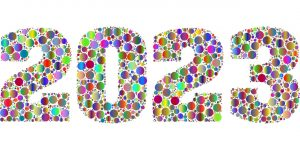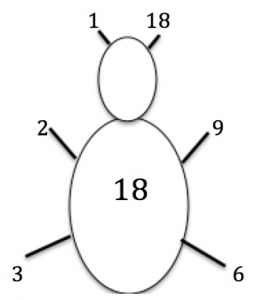Find the prime factors of 2023.

You can be a creative mathematician, you can be someone who has your own mathematical ideas. Find your own interesting facts about 2023 and calculations that have the answer 2023.

Write 2023 as a product of its prime factors.Here is the factor bug for 18.

The antennae show 1 × 18 = 18.

The pairs of legs show and 2 × 9 = 18 and 3 × 6 = 18.

Factor bugs for other numbers can have more legs.

Draw the factor bug for 2023. How many legs does it have?

How many ways can you arrange the digits 2, 2, 0 and 3 to get different numbers? What is the sum of those numbers?

Is it correct to say “twenty twenty-three” or should we say “two thousand and twenty-three” or are both correct?  Why?

People say “twenty twenty three” so do the 2 twenties mean – 20 thousands, 20 hundreds, 20 tens or 20 units?

What different questions can you find with 2023 as the answer?

Compare your questions with other people’s.

Perhaps you can make up one that is easy, one that is harder and one that is very hard.

What interesting facts can you find about the year that you were born?